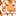# QlikView App Dev

Discussion Board for collaboration related to QlikView App Development.

cancel
Showing results for
Did you mean:Creator

## Set expression with variables

Here are the variables:

• vYearWeekLY_YD
• vWeekDayNumberYD

I'd like to turn this statement into a set expression:

Sum ( If( [Year Week] = vYearWeekLY_YD and WeekDayNumber = vWeekDayNumberYD, Fsgexkl_Loc*Comparable_week))

But when I do it like:

Sum ( {\$< [Year Week] ={'\$(=\$(vYearWeekLY_YD))'}, WeekDayNumber = {'\$(=\$(vWeekDayNumberYD))'} >} Fsgexkl_Loc*Comparable_week))

It does not work.

Where am I going wrong with this? It's always the same when dabbling with variables in set expressions - frustrating.

Thanks

5 RepliesContributor II

Try this

Sum ( {\$< [Year Week] ={"\$(vYearWeekLY_YD)"}, WeekDayNumber = {'\$(vWeekDayNumberYD)"} >} Fsgexkl_Loc*Comparable_week))MVP

Do you have a sample app to support and show case your issue?Creator
Author

I looked in our script and here's how the variables are created:

LET VECKANR_YD = num(Week(today()-1),00); //Week number yesterday

LET vYearWeek_YD = Year(Today()-1)&'W'& \$(VECKANR_YD); //year week yesterday

LET vYearWeekLY_YD = Year(AddMonths(Today()-1,-12))&'W'& \$(VECKANR_YD); //year week last year yesterdayContributor II

Try below :

Sum ( {\$  < [Year Week] ={\$(=vYearWeekLY_YD)}, WeekDayNumber = {\$(=vWeekDayNumberYD)} >  }   Fsgexkl_Loc*Comparable_week )Contributor II

Sum ( {\$  < [Year Week] ={'\$(vYearWeekLY_YD)'}, WeekDayNumber = {'\$(vWeekDayNumberYD)'} >  }   Fsgexkl_Loc*Comparable_week )